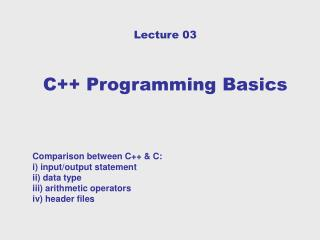# Lecture 03 C++ Programming Basics - PowerPoint PPT PresentationDownload PresentationLecture 03 C++ Programming Basics

Lecture 03 C++ Programming BasicsDownload Presentation## Lecture 03 C++ Programming Basics

- - - - - - - - - - - - - - - - - - - - - - - - - - - E N D - - - - - - - - - - - - - - - - - - - - - - - - - - -
##### Presentation Transcript

1. Lecture 03C++ Programming Basics Comparison between C++ & C:i) input/output statementii) data typeiii) arithmetic operatorsiv) header files

2. C++ vs C • C++ is a superset of C. • C++ was originally called “C with classes”.

3. Basic C++ Program Construct

4. Basic C++ Program Construct A Sample Program // first.cpp #include <iostream> //preprocessor using namespace std; //directive int main() { int ftemp; // temperature in Fahrenheit cout << "Enter temperature in Fahr.: "; cin >> ftemp; int ctemp = (ftemp - 32) * 5 / 9; cout << "Equivalent in Celsius is: " << ctemp << endl; return 0; }

6. Preprocessor Directive#include C Style: #include<stdio.h> C++ Style: #include<iostream.h> OR THE FOLLOWING ANSI STANDARD #include<iostream> using namespace std;

7. Input and Output C Input and Ouput: #include<stdio.h> scanf(“%i”, &num); printf(“The number is %i\n“, num); C++ Input and Ouput: #include<iostream.h> cin >> num; cout << “The number is “, num << endl;

8. Stream I/OInsertion and Extraction

9. Constants C-Style Symbolic Constant: #define PI 3.14159 We can’t specify the data type! C++ const Qualifier: const float PI = 3.14159; Recommended ! What is a Variable ? What is an Identifier? Rules for declaring an identifier? Int Sum1 ;Float 1Salaray

10. Boolean Type • Integer, character, and floating-point types are available both in C and C++. • Boolean type is available in C++, but not in C. • Variables of typebool can have only two possible values: true and false (Boolean Values). • Even though a single bit is enough to store variables of type bool, compilers often store them as integers for ease of processing.

11. Boolean Type Void Main( ) { int num; bool flag = true; Cin>> num; if (num > 0 ) flag = true; else flag = false; if (flag == true ) cout<<“positive number”; else cout “negative number”; } void main() { bool flag = true; if (flag) cout<<“Hello Halizah”<<endl; else cout<<“Hello World!”<<endl; }

12. Type Conversion : Order of Data Types

13. Automatic Type Conversion • When two operands of different types are encountered in the same expression, the lower-type variable is automatically converted to the higher type variable. int denominator; float numerator, quotient; ... quotient = numerator/denominator; • denominatoris converted from int to float before the division.

14. The setw Manipulator #include <iostream.h> #include <iomanip.h> // for setw int main() { long pop=2425785; cout << setw(8) << "LOCATION" << setw(12) << "POPULATION" << endl << setw(8) << "Portcity" << setw(12) << pop << endl return 0; } Output of the program: LOCATION POPULATION Portcity2425785

15. The <cmath> Standard Library Functions // sqrt.cpp // demonstrate sqrt() library function #include <iostream> // for cout, etc. #include <cmath> // for sqrt() using namespace std; int main() { double number, answer; cout << "Enter a number: "; cin >> number; answer = sqrt(number); cout << "Square root is " << answer << endl; return 0; }